The REG Procedure

MODEL Statement

• <label:> MODEL dependents = <regressors> </ options>;

After the keyword MODEL, the dependent (response) variables are specified, followed by an equal sign and the regressor variables. Variables specified in the MODEL statement must be numeric variables in the data set being analyzed. For example, if you want to specify a quadratic term for variable X1 in the model, you cannot use X1*X1 in the MODEL statement but must create a new variable (for example, X1SQUARE=X1*X1) in a DATA step and use this new variable in the MODEL statement. The label in the MODEL statement is optional.

Table 97.5 summarizes the options available in the MODEL statement. Equations for the statistics available are given in the section Model Fit and Diagnostic Statistics.

Table 97.5: MODEL Statement Options

Option

Description

Model Selection and Details of Selection

Specifies model selection method

Specifies maximum number of subset models displayed or output to the OUTEST= data set

Produces summary statistics at each step

Specifies the display details for FORWARD, BACKWARD, and STEPWISE methods

Provides names for groups of variables

Includes first n variables in the model

Specifies maximum number of steps that might be performed

Fits a model without the intercept term

Performs incomplete principal component analysis and outputs estimates to the OUTEST= data set

Performs ridge regression analysis and outputs estimates to the OUTEST= data set

Sets criterion for entry into model

Sets criterion for staying in model

Specifies number of variables in model to begin the comparing and switching process

Stops selection criterion

Statistics

Computes Akaike’s information criterion

Computes parameter estimates for each model

Computes Sawa’s Bayesian information criterion

Computes Mallows’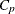statistic

Computes estimated MSE of prediction assuming multivariate normality

Computes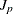, the final prediction error

Computes MSE for each model

Computes Amemiya’s prediction criterion

Displays root MSE for each model

Computes the SBC statistic

Computes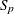statistic for each model

Computes error sum of squares for each model

Data Set Options

Outputs the number of regressors, the error degrees of freedom, and the model R square to the OUTEST= data set

Outputs standard errors of the parameter estimates to the OUTEST= data set

Outputs standardized parameter estimates to the OUTEST= data set. Use only with the RIDGE= or PCOMIT= option.

Outputs the variance inflation factors to the OUTEST= data set. Use only with the RIDGE= or PCOMIT= option.

Outputs the PRESS statistic to the OUTEST= data set

Has same effect as the EDF option

Regression Calculations

Displays inverse of sums of squares and crossproducts

Displays sums-of-squares and crossproducts matrix

Details on Estimates

Displays heteroscedasticity- consistent covariance matrix of estimates and heteroscedasticity-consistent standard errors

Specifies method for computing the asymptotic heteroscedasticity-consistent covariance matrix

Produces collinearity analysis

Produces collinearity analysis with intercept adjusted out

Displays correlation matrix of estimates

Displays covariance matrix of estimates

Displays heteroscedasticity-consistent standard errors

Specifies method for computing the asymptotic heteroscedasticity-consistent covariance matrix

Performs lack-of-fit test

Displays squared semipartial correlation coefficients computed using Type I sums of squares

Displays squared partial correlation coefficients computed using Type I sums of squares

Displays squared partial correlation coefficients computed using Type II sums of squares

Displays squared semipartial correlation coefficients computed using Type I sums of squares

Displays squared semipartial correlation coefficients computed using Type II sums of squares

Displays a sequence of parameter estimates during selection process

Tests that first and second moments of model are correctly specified

Displays the studentized residuals by using a series of asterisks

Displays the sequential sums of squares

Displays the partial sums of squares

Displays standardized parameter estimates

Displays tolerance values for parameter estimates

Displays heteroscedasticity-consistent standard errors

Computes variance-inflation factors

Predicted and Residual Values

Computes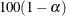% confidence limits for the parameter estimates

Computes% confidence limits for an individual predicted value

Computes% confidence limits for the expected value of the dependent variable

Computes a Durbin-Watson statistic

Computes a Durbin-Watson statistic and p-value

Computes influence statistics

Computes predicted values

Displays partial regression plots for each regressor

Displays partial regression data

Produces analysis of residuals

Display Options and Other Options

Requests the following options:
ACOV , CLB , CLI , CLM , CORRB , COVB , HCC , I , P ,
PCORR1 , PCORR2 , R , SCORR1 , SCORR2 , SEQB , SPEC ,
SS1 , SS2 , STB , TOL , VIF , XPX

Sets significance value for confidence and prediction intervals and tests

Suppresses display of results

Specifies the true standard deviation of error term for computing CP and BIC

Sets criterion for checking for singularity

You can specify the following options in the MODEL statement after a slash (/).

ACOV

displays the estimated asymptotic covariance matrix of the estimates under the hypothesis of heteroscedasticity and heteroscedasticity-consistent standard errors of parameter estimates. See the HCCMETHOD= option and the HCC option and the section Testing for Heteroscedasticity for more information.

ACOVMETHOD=0,1,2, or 3

See the HCCMETHOD= option.

computes R square adjusted for degrees of freedom for each model selected (Darlington 1968; Judge et al. 1980).

AIC

outputs Akaike’s information criterion for each model selected (Akaike 1969; Judge et al. 1980) to the OUTEST= data set. If SELECTION=ADJRSQ, SELECTION=RSQUARE, or SELECTION=CP is specified, then the AIC statistic is also added to the SubsetSelSummary table.

ALL

requests all these options: ACOV, CLB, CLI, CLM, CORRB, COVB, HCC, I, P, PCORR1, PCORR2, R, SCORR1, SCORR2, SEQB, SPEC, SS1, SS2, STB, TOL, VIF, and XPX.

ALPHA=number

sets the significance level used for the construction of confidence intervals for the current MODEL statement. The value must be between 0 and 1; the default value of 0.05 results in 95% intervals. This option affects the MODEL options CLB, CLI, and CLM; the OUTPUT statement keywords LCL, LCLM, UCL, and UCLM; the PLOT statement keywords LCL., LCLM., UCL., and UCLM.; and the PLOT statement options CONF and PRED. If you specify this option in the MODEL statement, it takes precedence over the ALPHA= option in the PROC REG statement.

B

is used with the RSQUARE, ADJRSQ, and CP model-selection methods to compute estimated regression coefficients for each model selected.

BEST=n

is used with the RSQUARE, ADJRSQ, and CP model-selection methods. If SELECTION=CP or SELECTION=ADJRSQ is specified, the BEST= option specifies the maximum number of subset models to be displayed or output to the OUTEST= data set. For SELECTION=RSQUARE, the BEST= option requests the maximum number of subset models for each size.

If the BEST= option is used without the B option (displaying estimated regression coefficients), the variables in each MODEL are listed in order of inclusion instead of the order in which they appear in the MODEL statement.

If the BEST= option is omitted and the number of regressors is less than 11, all possible subsets are evaluated. If the BEST= option is omitted and the number of regressors is greater than 10, the number of subsets selected is, at most, equal to the number of regressors. A small value of the BEST= option greatly reduces the CPU time required for large problems.

BIC

outputs Sawa’s Bayesian information criterion for each model selected (Sawa 1978; Judge et al. 1980) to the OUTEST= data set. If SELECTION=ADJRSQ, SELECTION=RSQUARE, or SELECTION=CP is specified, then the BIC statistic is also added to the SubsetSelSummary table.

CLB

requests the% upper and lower confidence limits for the parameter estimates. By default, the 95% limits are computed; the ALPHA= option in the PROC REG or MODEL statement can be used to change thelevel. If any of the MODEL statement options ACOV , HCC , or WHITE are in effect, then the CLB option also produces heteroscedasticity-consistent% upper and lower confidence limits for the parameter estimates.

CLI

requests the% upper and lower confidence limits for an individual predicted value. By default, the 95% limits are computed; the ALPHA= option in the PROC REG or MODEL statement can be used to change thelevel. The confidence limits reflect variation in the error, as well as variation in the parameter estimates. See the section Predicted and Residual Values and Chapter 4: Introduction to Regression Procedures, for more information.

CLM

displays the% upper and lower confidence limits for the expected value of the dependent variable (mean) for each observation. By default, the 95% limits are computed; the ALPHA= in the PROC REG or MODEL statement can be used to change thelevel. This is not a prediction interval (see the CLI option) because it takes into account only the variation in the parameter estimates, not the variation in the error term. See the section Predicted and Residual Values and Chapter 4: Introduction to Regression Procedures, for more information.

COLLIN

requests a detailed analysis of collinearity among the regressors. This includes eigenvalues, condition indices, and decomposition of the variances of the estimates with respect to each eigenvalue. See the section Collinearity Diagnostics.

COLLINOINT

requests the same analysis as the COLLIN option with the intercept variable adjusted out rather than included in the diagnostics. See the section Collinearity Diagnostics.

CORRB

displays the correlation matrix of the estimates. This is the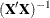matrix scaled to unit diagonals.

COVB

displays the estimated covariance matrix of the estimates. This matrix is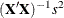, where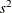is the estimated mean squared error.

CP

outputs Mallows’statistic for each model selected (Mallows 1973; Hocking 1976) to the OUTEST= data set. See the section Criteria Used in Model-Selection Methods for a discussion of the use of. If SELECTION=ADJRSQ, SELECTION=RSQUARE, or SELECTION=CP is specified, then thestatistic is also added to the SubsetSelSummary table.

DETAILS
DETAILS=name

specifies the level of detail produced when the BACKWARD, FORWARD, or STEPWISE method is used, where name can be ALL, STEPS, or SUMMARY. The DETAILS or DETAILS=ALL option produces entry and removal statistics for each variable in the model building process, ANOVA and parameter estimates at each step, and a selection summary table. The option DETAILS=STEPS provides the step information and summary table. The option DETAILS=SUMMARY produces only the summary table. The default if the DETAILS option is omitted is DETAILS=STEPS.

DW

calculates a Durbin-Watson statistic to test whether or not the errors have first-order autocorrelation. (This test is appropriate only for time series data.) Note that your data should be sorted by the date/time ID variable before you use this option. The sample autocorrelation of the residuals is also produced. See the section Autocorrelation in Time Series Data.

DWPROB

calculates a Durbin-Watson statistic and a p-value to test whether or not the errors have first-order autocorrelation. Note that it is not necessary to specify the DW option if the DWPROB option is specified. (This test is appropriate only for time series data.) Note that your data should be sorted by the date/time ID variable before you use this option. The sample autocorrelation of the residuals is also produced. See the section Autocorrelation in Time Series Data.

EDF

outputs the number of regressors in the model excluding and including the intercept, the error degrees of freedom, and the model R square to the OUTEST= data set.

GMSEP

outputs the estimated mean square error of prediction assuming that both independent and dependent variables are multivariate normal (Stein 1960; Darlington 1968) to the OUTEST= data set. (Note that Hocking’s formula (1976, eq. 4.20) contains a misprint: "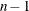" should read "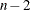.") If SELECTION=ADJRSQ, SELECTION=RSQUARE, or SELECTION=CP is specified, then the GMSEP statistic is also added to the SubsetSelSummary table.

GROUPNAMES=’name1’ ’name2’ …

provides names for variable groups. This option is available only in the BACKWARD, FORWARD, and STEPWISE methods. The group name can be up to 32 characters. Subsets of independent variables listed in the MODEL statement can be designated as variable groups. This is done by enclosing the appropriate variables in braces. Variables in the same group are entered into or removed from the regression model at the same time. However, if the tolerance of any variable (see the TOL option) in a group is less than the setting of the SINGULAR= option, then the variable is not entered into the model with the rest of its group. If the GROUPNAMES= option is not used, then the names GROUP1, GROUP2, …, GROUPn are assigned to groups encountered in the MODEL statement. Variables not enclosed by braces are used as groups of a single variable.

For example:

model y={x1 x2} x3 / selection=stepwise
groupnames='x1 x2' 'x3';


Another example:

model y={ht wgt age} bodyfat / selection=forward
groupnames='htwgtage' 'bodyfat';

HCC

requests heteroscedasticity-consistent standard errors of the parameter estimates. You can use the HCCMETHOD= option to specify the method used to compute the heteroscedasticity-consistent covariance matrix.

HCCMETHOD=0,1,2, or 3

specifies the method used to obtain a heteroscedasticity-consistent covariance matrix for use with the ACOV , HCC , or WHITE option in the MODEL statement and for heteroscedasticity-consistent tests with the TEST statement. The default is HCCMETHOD=0. See the section Testing for Heteroscedasticity for details.

I

displays the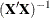matrix. The inverse of the crossproducts matrix is bordered by the parameter estimates and SSE matrices.

INCLUDE=n

forces the first n independent variables listed in the MODEL statement to be included in all models. The selection methods are performed on the other variables in the MODEL statement. The INCLUDE= option is not available with SELECTION=NONE.

INFLUENCE

requests a detailed analysis of the influence of each observation on the estimates and the predicted values. See the section Influence Statistics for details.

JP

outputs, the estimated mean square error of prediction for each model selected assuming that the values of the regressors are fixed and that the model is correct to the OUTEST= data set. Thestatistic is also called the final prediction error (FPE) by Akaike (Nicholson 1948; Lord 1950; Mallows 1967; Darlington 1968; Rothman 1968; Akaike 1969; Hocking 1976; Judge et al. 1980) If SELECTION=ADJRSQ, SELECTION=RSQUARE, or SELECTION=CP is specified, then thestatistic is also added to the SubsetSelSummary table.

LACKFIT

performs a lack-of-fit test. See the section Testing for Lack of Fit for more information. See Draper and Smith (1981) for a discussion of lack-of-fit tests.

MSE

computes the mean square error for each model selected (Darlington 1968).

MAXSTEP=n

specifies the maximum number of steps that are done when SELECTION=FORWARD, SELECTION=BACKWARD, or SELECTION=STEPWISE is used. The default value is the number of independent variables in the model for the FORWARD and BACKWARD methods and three times this number for the stepwise method.

NOINT

suppresses the intercept term that is otherwise included in the model.

NOPRINT

suppresses the normal display of regression results. Note that this option temporarily disables the Output Delivery System (ODS); see Chapter 20: Using the Output Delivery System, for more information.

OUTSEB

outputs the standard errors of the parameter estimates to the OUTEST= data set. The value SEB for the variable _TYPE_ identifies the standard errors. If the RIDGE= or PCOMIT= option is specified, additional observations are included and identified by the values RIDGESEB and IPCSEB, respectively, for the variable _TYPE_. The standard errors for ridge regression estimates and incomplete principal components (IPC) estimates are limited in their usefulness because these estimates are biased. This option is available for all model-selection methods except RSQUARE, ADJRSQ, and CP.

OUTSTB

outputs the standardized parameter estimates as well as the usual estimates to the OUTEST= data set when the RIDGE= or PCOMIT= option is specified. The values RIDGESTB and IPCSTB for the variable _TYPE_ identify ridge regression estimates and IPC estimates, respectively.

OUTVIF

outputs the variance inflation factors (VIF) to the OUTEST= data set when the RIDGE= or PCOMIT= option is specified. The factors are the diagonal elements of the inverse of the correlation matrix of regressors as adjusted by ridge regression or IPC analysis. These observations are identified in the output data set by the values RIDGEVIF and IPCVIF for the variable _TYPE_.

P

calculates predicted values from the input data and the estimated model. The display includes the observation number, the ID variable (if one is specified), the actual and predicted values, and the residual. If the CLI, CLM, or R option is specified, the P option is unnecessary. See the section Predicted and Residual Values for more information.

PARTIAL

requests partial regression leverage plots for each regressor. You can use the PARTIALDATA option to obtain a tabular display of the partial regression leverage data. If ODS Graphics is enabled (see the section ODS Graphics), then these partial plots are produced in panels with up to six plots per panel. See the section Influence Statistics for more information.

PARTIALDATA

requests partial regression leverage data for each regressor. You can request partial regression leverage plots of these data with the PARTIAL option. See the section Influence Statistics for more information.

PARTIALR2 <( < TESTS> <SEQTESTS> ) >

See the SCORR1 option.

PC

outputs Amemiya’s prediction criterion for each model selected (Amemiya 1976; Judge et al. 1980) to the OUTEST= data set. If SELECTION=ADJRSQ, SELECTION=RSQUARE, or SELECTION=CP is specified, then the PC statistic is also added to the SubsetSelSummary table.

PCOMIT=list

requests an IPC analysis for each value m in the list. The procedure computes parameter estimates by using all but the last m principal components. Each value of m produces a set of IPC estimates, which is output to the OUTEST= data set. The values of m are saved by the variable _PCOMIT_, and the value of the variable _TYPE_ is set to IPC to identify the estimates. Only nonnegative integers can be specified with the PCOMIT= option.

If you specify the PCOMIT= option, RESTRICT statements are ignored. The PCOMIT= option is ignored if you use the SELECTION= option in the MODEL statement.

PCORR1

displays the squared partial correlation coefficients computed using Type I sum of squares (SS). This is calculated as SS/(SS+SSE), where SSE is the error sum of squares.

PCORR2

displays the squared partial correlation coefficients computed using Type II sums of squares. These are calculated the same way as with the PCORR1 option, except that Type II SS are used instead of Type I SS.

PRESS

outputs the PRESS statistic to the OUTEST= data set. The values of this statistic are saved in the variable _PRESS_. This option is available for all model-selection methods except RSQUARE, ADJRSQ, and CP.

R

requests an analysis of the residuals. The results include everything requested by the P option plus the standard errors of the mean predicted and residual values, the studentized residual, and Cook’s D statistic to measure the influence of each observation on the parameter estimates. See the section Predicted and Residual Values for more information.

RIDGE=list

requests a ridge regression analysis and specifies the values of the ridge constant k (see the section Computations for Ridge Regression and IPC Analysis). Each value of k produces a set of ridge regression estimates that are placed in the OUTEST= data set. The values of k are saved by the variable _RIDGE_, and the value of the variable _TYPE_ is set to RIDGE to identify the estimates.

Only nonnegative numbers can be specified with the RIDGE= option. Example 97.5 illustrates this option.

If you specify the RIDGE= option, RESTRICT statements are ignored. The RIDGE= option is ignored if you use the SELECTION= option in the MODEL statement.

RMSE

displays the root mean square error for each model selected.

RSQUARE

has the same effect as the EDF option.

SBC

outputs the SBC statistic for each model selected (Schwarz 1978; Judge et al. 1980) to the OUTEST= data set. If SELECTION=ADJRSQ, SELECTION=RSQUARE, or SELECTION=CP is specified, then the SBC statistic is also added to the SubsetSelSummary table.

SCORR1 <( < TESTS> <SEQTESTS> ) >

displays the squared semipartial correlation coefficients computed using Type I sums of squares. This is calculated as SS/SST, where SST is the corrected total SS. If the NOINT option is used, the uncorrected total SS is used in the denominator. The optional arguments TESTS and SEQTESTS request are sequentially added to a model. The F-test values are computed as the Type I sum of squares for the variable in question divided by a mean square error. If you specify the TESTS option, the denominator MSE is the residual mean square for the full model specified in the MODEL statement. If you specify the SEQTESTS option, the denominator MSE is the residual mean square for the model containing all the independent variables that have been added to the model up to and including the variable in question. The TESTS and SEQTESTS options are not supported if you specify model selection methods or the RIDGE or PCOMIT options. Note that the PARTIALR2 option is a synonym for the SCORR1 option.

SCORR2 <( TESTS )>

displays the squared semipartial correlation coefficients computed using Type II sums of squares. These are calculated the same way as with the SCORR1 option, except that Type II SS are used instead of Type I SS. The optional TEST argument requests F tests and p-values as variables are sequentially added to a model. The F-test values are computed as the Type II sum of squares for the variable in question divided by the residual mean square for the full model specified in the MODEL statement. The TESTS option is not supported if you specify model selection methods or the RIDGE or PCOMIT options.

SELECTION=name

specifies the method used to select the model, where name can be FORWARD (or F), BACKWARD (or B), STEPWISE, MAXR, MINR, RSQUARE, ADJRSQ, CP, or NONE (use the full model). The default method is NONE. See the section Model-Selection Methods for a description of each method.

SEQB

produces a sequence of parameter estimates as each variable is entered into the model. This is displayed as a matrix where each row is a set of parameter estimates.

SIGMA=n

specifies the true standard deviation of the error term to be used in computing the CP and BIC statistics. If the SIGMA= option is not specified, an estimate from the full model is used. This option is available in the RSQUARE, ADJRSQ, and CP model-selection methods only.

SINGULAR=n

tunes the mechanism used to check for singularities. If you specify this option in the MODEL statement, it takes precedence over the SINGULAR= option in the PROC REG statement. The default value is machine dependent but is approximately 1E–7 on most machines. This option is rarely needed. Singularity checking is described in the section Computational Methods.

SLENTRY=value
SLE=value

specifies the significance level for entry into the model used in the FORWARD and STEPWISE methods. The defaults are 0.50 for FORWARD and 0.15 for STEPWISE.

SLSTAY=value
SLS=value

specifies the significance level for staying in the model for the BACKWARD and STEPWISE methods. The defaults are 0.10 for BACKWARD and 0.15 for STEPWISE.

SP

outputs thestatistic for each model selected (Hocking 1976) to the OUTEST= data set. If SELECTION=ADJRSQ, SELECTION=RSQUARE, or SELECTION=CP is specified, then the SP statistic is also added to the SubsetSelSummary table.

SPEC

performs a test that the first and second moments of the model are correctly specified. See the section Testing for Heteroscedasticity for more information.

SRT

displays a column in the "Output Statistics" table that shows the magnitude of studentized residuals by using a series of asterisks. This table is produced by the R option in the MODEL statement. When ODS Graphics is not enabled, the column is displayed in the table and the graph is not produced. When ODS Graphics is enabled, a graphical chart of the studentized residuals and Cook’s D is produced, and the column is omitted from the table unless you specify the SRT option.

SS1

displays the sequential sums of squares (Type I SS) along with the parameter estimates for each term in the model. See Chapter 15: The Four Types of Estimable Functions, for more information about the different types of sums of squares.

SS2

displays the partial sums of squares (Type II SS) along with the parameter estimates for each term in the model. See the SS1 option also.

SSE

computes the error sum of squares for each model selected.

START=s

specifies the number of regressors to be added or reported in a model.

When SELECTION= MAXR, MINR, or STEPWISE, START=s requests that the first s regressors be added to the model before beginning the comparing-and-switching process. Thus, START=s behaves like INCLUDE= s in that it forces selection to begin with the first s regressors included in the model, but it differs in that START=s allows these variables to be subsequently removed from the model in the course of variable selection. For these methods, the default is START=0.

When SELECTION= RSQUARE, ADJRSQ, or CP, START=s specifies the smallest number of regressors to be reported in a subset model. For these methods, the default is START=1.

The START= option cannot be used with model-selection methods other than the six described here.

STB

produces standardized regression coefficients. A standardized regression coefficient is computed by dividing a parameter estimate by the ratio of the sample standard deviation of the dependent variable to the sample standard deviation of the regressor.

STOP=s

causes PROC REG to stop when it has found the "best" s-variable model, where s is the STOP value. For the RSQUARE, ADJRSQ, and CP methods, STOP=s specifies the largest number of regressors to be reported in a subset model. For the MAXR and MINR methods, STOP=s specifies the largest number of regressors to be included in the model.

The default setting for the STOP= option is the number of variables in the MODEL statement. This option can be used only with the MAXR, MINR, RSQUARE, ADJRSQ, and CP methods.

TOL

produces tolerance values for the estimates. Tolerance for a variable is defined as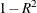, where R square is obtained from the regression of the variable on all other regressors in the model. See the section Collinearity Diagnostics for more details.

VIF

produces variance inflation factors with the parameter estimates. Variance inflation is the reciprocal of tolerance. See the section Collinearity Diagnostics for more detail.

WHITE

See the HCC option.

XPX

displays thecrossproducts matrix for the model. The crossproducts matrix is bordered by the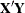and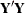matrices.